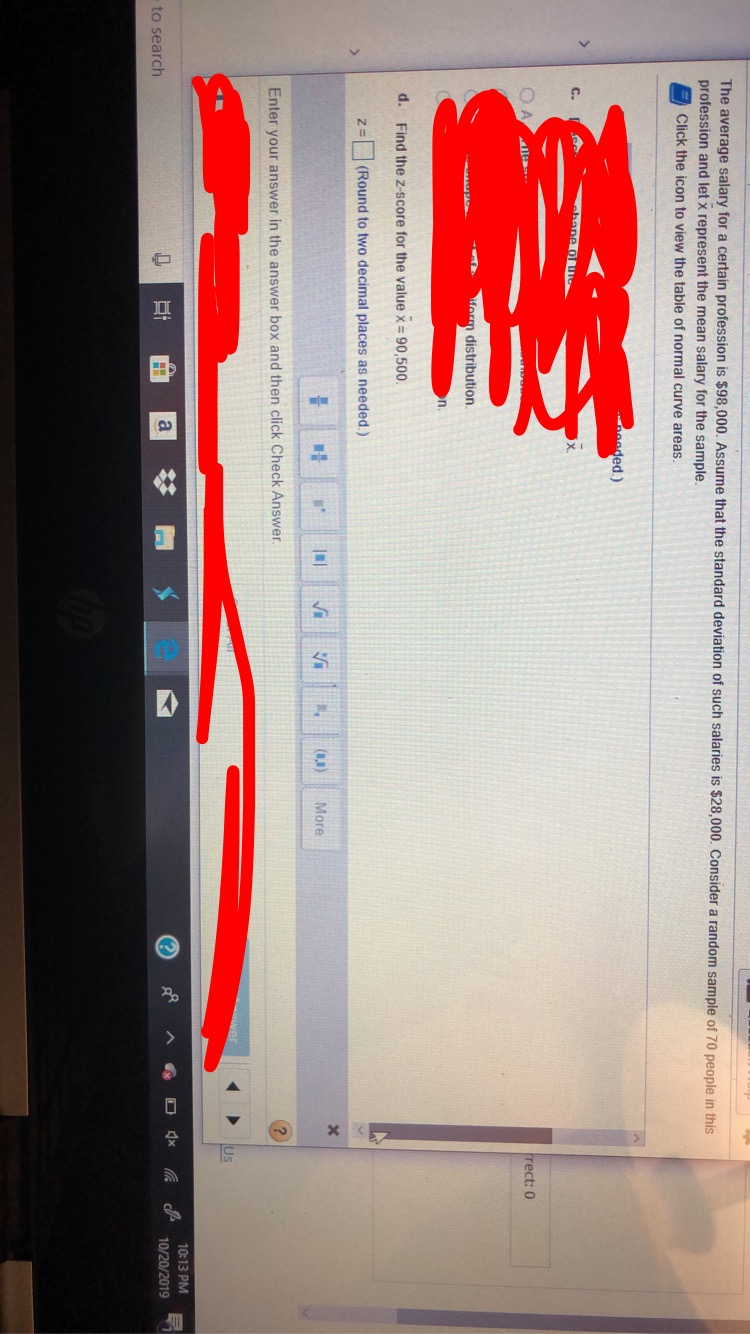# The average salary for a certain profession is \$98,000. Assume that the standard deviation of such salaries is \$28,000. Consider a random sample of 70 people in thisprofession and let x represent the mean salary for the sample.Click the icon to view the table of normal curve areas.ded.)bane OlC.rect: 0iform distribution.n.d.Find the z-score for the value x90,500.(Round to two decimal places as needed.)MoreEnter your answer in the answer box and then click Check AnswerUs10:13 PM10/20/2019to search

Question

Please show steps for TI 84 as well.help_outlineImage TranscriptioncloseThe average salary for a certain profession is \$98,000. Assume that the standard deviation of such salaries is \$28,000. Consider a random sample of 70 people in this profession and let x represent the mean salary for the sample. Click the icon to view the table of normal curve areas. ded.) bane Ol C. rect: 0 iform distribution. n. d. Find the z-score for the value x 90,500. (Round to two decimal places as needed.) More Enter your answer in the answer box and then click Check Answer Us 10:13 PM 10/20/2019 to search fullscreen
check_circleExpert Solution
Step 1

Introduction:

Suppose a random variable X has mean μ and standard deviation, σ. Then, the z-score corresponding to a particular value, X = x is:

z = (xμ)/σ.

For a sample mean value, the expected value is μ = μ and standard deviation σ = σ/&...

### Want to see the full answer?

See Solution

#### Want to see this answer and more?

Solutions are written by subject experts who are available 24/7. Questions are typically answered within 1 hour*

See Solution
*Response times may vary by subject and question
Tagged in

### Other